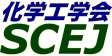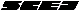## \$B9V1i%W%m%0%i%`!J2q>l!&F|DxJL!K(B

### Q\$B2q>l(B \$BBh(B3\$BF|(B

\$B:G=*99?7F|;~!'(B2012-08-20 15:02:17
\$B9V1i(B
\$B;~9o(B
\$B9V1i(B
\$BHV9f(B
\$B9V1iBjL\!?H/I=\$B%-!<%o!<%I(B\$BJ,N`(B
\$BHV9f(B
\$B\$BHV9f(B
\$B%7%s%]%8%&%`(B \$B!c4D6-It2q%7%s%]%8%&%`!d(B
(9:20\$B!A(B10:20)\$B!!(B(\$B:BD9(B \$B>.6L(B \$BAo(B)
9:20\$B!A(B 9:40Q302\$BB?9&
(\$B?.=#BgA!0](B) \$B!{(B(\$B3X(B)\$B? \$B!&(B (\$B@5(B)\$B9b66(B \$B?-1Q(B \$B!&(B (\$B@5(B)\$BJ!D9(B \$BGn(B \$B!&(B (\$B@5(B)\$B9bDM(B \$BF)(B
CO2 chemical absorption
hollow fiber membrane contactor
cross-flow module
S-10998
9:40\$B!A(B 10:00Q303\$BM-5!B?9&
(\$B?.=#BgA!0](B) \$B!{(B(\$B3X(B)\$BOBED(B \$BBsLi(B \$B!&(B (\$B@5(B)\$B9b66(B \$B?-1Q(B \$B!&(B (\$B@5(B)\$BJ!D9(B \$BGn(B \$B!&(B (\$B@5(B)\$B9bDM(B \$BF)(B
CO2 chemical absorption
microporous hollow fiber membrane
absorbent regeneration
S-10999
10:00\$B!A(B 10:20Q304\$B%;%i%_%C%/%95[<}:`=2\$B5[<}NL\$NI>2A(B
(\$BAaBg1!(B) \$B!{(B(\$B3X(B)\$BEDCf(B \$B5.9I(B \$B!&(B (\$B@5(B)\$BCf3@(B \$BN4M:(B \$B!&(B \$BEDH*(B \$B1M \$B!&(B (NTT) \$B@wB<(B \$BMG(B \$B!&(B \$B4]Hx(B \$BMF;R(B \$B!&(B \$B?e>B(B \$B
Lithium Silicate
CO2 Absorbent
Heat of Absorption
S-10217
(10:20\$B!A(B11:00)\$B!!(B(\$B:BD9(B \$B9b66(B \$B?-1Q(B)
10:20\$B!A(B 10:40Q305TSA\$BJ}<0\$rMQ\$\$\$?G3>FGS%,%9\$+\$i\$N(BCO2\$BJ,N%!&G;=L!&2s<}%7%9%F%`\$N3+H/(B
(\$B@>It5;8&(B) \$B!{(B(\$B@5(B)\$B0f>e(B \$B9(;V(B \$B!&(B (\$B@5(B)\$B4];3(B \$B9aL>9>(B \$B!&(B (\$B@5(B)\$B2,Ln(B \$B9@;V(B
honeycomb rotor
CO2 concentration and recovery
S-10536
10:40\$B!A(B 11:00Q306\$B@PC:2PNOH/EE=jGS%,%9\$KBP\$9\$k(BCO2\$BJ,N%2s<}5;=Q\$N%W%m%;%9Hf3S(B
(\$BEl9)Bg1!9)(B) \$B!{(B(\$B@5(B)\$B>.6L(B \$BAo(B \$B!&(B (RITE) (\$B@5(B)\$B8eF#(B \$BOBLi(B \$B!&(B (\$BEl9)Bg1!9)(B) (\$B@5(B)\$B4X8}(B \$B=(=S(B
CO2
Carbon Capture
Process Simulation
S-10778
(11:00\$B!A(B12:00)\$B!!(B(\$B;J2q(B \$BEDCf(B \$B44Li(B)
11:00\$B!A(B 12:00Q307[\$BE8K>9V1i(B] \$B%l%"%a%?%k%j%5%\$%/%k\$NF08~(B
(\$BElKLBg(B) \$B!{CfB<(B \$B?r(B
rare metals
recycling
metallurgy
S-10539
(13:00\$B!A(B14:00)\$B!!(B(\$B:BD9(B \$B2#;3(B \$B8x0l(B)
13:00\$B!A(B 13:20Q313CO2\$B5[<}MQ%"%_%s?eMO1U\$NHfG.B,Dj(B
(\$BEl \$B!&(B (\$B@5(B)\$B>.@n(B \$BEM(B
Carbon Capture
Amine Solution
Specific Heat
S-1021
13:20\$B!A(B 13:40Q314CCS\$B%W%i%s%H\$+\$i\$NBg5\$J|;6%"%_%s\$N3H;65sF02r@O(B
(\$BEl \$B!&(B (\$B@5(B)\$BF#ED(B \$B8J;W?M(B \$B!&(B \$B1vED(B \$BOBB'(B \$B!&(B (\$B@5(B)\$BKLB<(B \$B1QIW(B
CCS
amine
emission
S-1078
13:40\$B!A(B 14:00Q315\$B%"%_%s5[<}1U>t2=%W%m%;%92r@O(B
(\$BEl \$B!&(B (\$B@5(B)\$BEZ20(B \$BD> \$B!&(B (\$B@5(B)\$BB<2,(B \$BBg8g(B \$B!&(B \$B1vED(B \$BOBB'(B \$B!&(B \$B:XF#(B \$BAo(B
CCS
amine
ion exchange resin
S-10189
(14:00\$B!A(B15:20)\$B!!(B(\$B:BD9(B \$BA0Bt(B \$B9,HK(B)
14:00\$B!A(B 14:20Q316\$B9bBQ5W@-%"%_%s5[<}1U\$rMQ\$\$\$?(BCO2\$B2=3X5[<}%W%m%;%9\$N3+H/(B
(\$B%P%VF|N)8b8&(B) \$B!{(B(\$B@5(B)\$B2#;3(B \$B8x0l(B \$B!&(B (\$B@5(B)\$B5\K\(B \$B1Q<#(B \$B!&(B \$B8|KQ(B \$BH~Jf(B \$B!&(B (\$B@5(B)\$B9bK\(B \$B@.?N(B \$B!&(B (\$B%P%VF|N)(B) \$BEgB<(B \$B=a(B \$B!&(B (\$B%P%VF|N)8b8&(B) (\$B@5(B)\$B>.NS(B \$BOB
Chemical Absorption
Carbon dioxide
Amine
S-10225
14:20\$B!A(B 14:40Q317\$B%b%N%(%?%N!<%k%"%_%sMO1U\$X\$NFs;@2=C:AG5[<}\$NB.EYO@E*8!F\$(B
(\$BL>Bg1!9)(B) \$B!{(B(\$B3X(B)\$B8w2,(B \$BD>Li(B \$B!&(B \$BDM86(B \$B>-;J(B \$B!&(B (\$B@5(B)\$B;{Lg(B \$B=\$(B \$B!&(B (\$B@5(B)\$BJ?_7(B \$B@/W"(B \$B!&(B (\$B%P%VF|N)8b8&(B) (\$B@5(B)\$B2#;3(B \$B8x0l(B \$B!&(B (\$B@5(B)\$B>.NS(B \$BOB
Post-Combustion Capture
Monoethanolamine
Carbon dioxide
S-10705
14:40\$B!A(B 15:00Q3182\$B@.J,7O:.9g5[<}1U\$K\$*\$1\$k(BCO2\$B5[<}B.EY\$KM?\$(\$k(BpH\$B\$N1F6A(B
(\$BAaBg1!(B) \$B!{(B(\$B3X(B)\$B=UED(B \$B9d;V(B \$B!&(B \$BB-N)(B \$BCR?.(B \$B!&(B (\$B@5(B)\$BCf3@(B \$BN4M:(B \$B!&(B \$B?7ED(B \$BH~Jf(B \$B!&(B \$B8E@n(B \$B9TIW(B \$B!&(B (IHI) \$B:4F#(B \$BM5(B
CCS
rate of absorption
amine
S-10690
15:00\$B!A(B 15:20Q3192\$B@.J,7O:.9g5[<}1U\$N(BCO2\$B5[<}H?1~G.\$K4X\$9\$k8&5f(B
(\$BAaBg1!(B) \$B!{(B(\$B3X(B)\$BEDCf(B \$B0lJ?(B \$B!&(B \$BHDAR(B \$BBs:H(B \$B!&(B (\$B@5(B)\$BCf3@(B \$BN4M:(B \$B!&(B \$B?7ED(B \$BH~Jf(B \$B!&(B \$B8E@n(B \$B9TIW(B \$B!&(B (IHI) \$B:4F#(B \$BM5(B
CCS
amine
heat of absorption
S-10769

\$B9V1i%W%m%0%i%`(B
\$B2=3X9)3X2q(B \$BBh(B44\$B2s=)5(Bg2q(B(C) 2012 \$B8x1W
Most recent update: 2012-08-20 15:02:17
For more information contact \$B2=3X9)3X2qElKL;YIt(B \$BBh(B44\$B2s=)5(Bg2q(B \$BLd\$\$9g\$;78(B
E-mail: inquiry-44fwww3.scej.org
This page was generated byeasp 2.28; proghtml 2.28c (C)1999-2011 kawase